# Ordinary Least-Squares with Measurement Error¶

## Unit 12, Lecture 4¶

Numerical Methods and Statistics

## Goals:¶

1. Be able to plot with error bars
2. Be able to choose the appropiate case for OLS with measurement error
3. Are aware of attenuation error and its effect on independent variable measurement error
In :
%matplotlib inline
import numpy as np
import matplotlib.pyplot as plt
from math import sqrt, pi, erf
import seaborn
seaborn.set_context("notebook")
seaborn.set_style("whitegrid")
import scipy.stats


# Plotting with Error Bars¶

Error bars are little lines that go up and down or left and right in graphs to indicate the standard error of a measurement. They may also indicate a confidence interval or standard deviation, however that will usually be specified in the figure caption. The code to make error bar plots is shown below with a constant y-error bar.

In :
x = np.array([1,2,4,4.5,7,10])
y = 3 * x + 2

#yerr=1 means constant standard error up and down of 1
#fmt is for lines/points and color. capthick is necessary to make the small dashes at the top and bottom
plt.errorbar(x,y, yerr=1, fmt='o', capthick=2)
plt.show()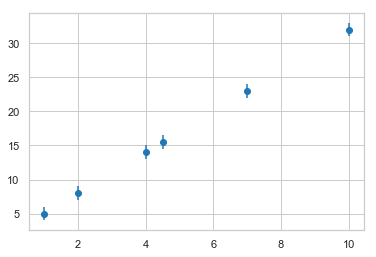Somtimes you have x-error and y-error. You can do that too:

In :
plt.errorbar(x,y, yerr=1, xerr=0.5, fmt='o', capthick=2)
plt.show()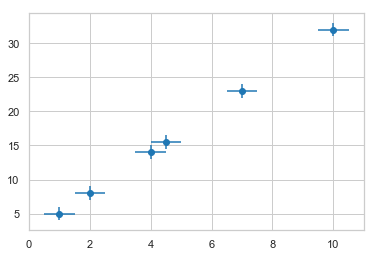You may have a different error value at each point. Then you pass an array instead:

In :
#THIS IS NOT PART OF PLOTTING ERROR BARS
#DO NOT COPY FOR HW/TEST
#THIS IS TO CREATE FAKE DATA FOR PLOTTING
#create some random numbers to use for my error bars
#squre them to make sure they're positive
yerror = 5*np.random.random(size=len(x))**2
#END

plt.errorbar(x,y, yerr=yerror, xerr=0.5, fmt='o', capthick=2)
plt.show()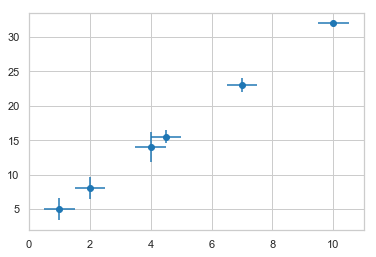If you do quantiling or some other technique that is non-parametric, you often can have error bars that are asymmetric. Then you need to pass in a 2xN array that has the distance up in the first row and distance down in the second row.

In :
#THIS IS NOT PART OF PLOTTING ERROR BARS
#DO NOT COPY FOR HW/TEST
#THIS IS TO CREATE FAKE DATA FOR PLOTTING
#create some random numbers to use for my error bars
#squre them to make sure they're positive
yerror_up = 2.5*np.random.random(size=len(x))**2
yerror_down = 2.5*np.random.random(size=len(x))**2
#END

yerror = np.row_stack( (yerror_up, yerror_down) )

plt.errorbar(x,y, yerr=yerror, xerr=0.5, fmt='o', capthick=2)
plt.show()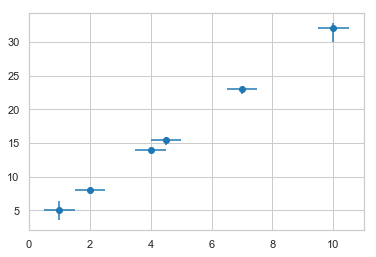# Ordinary Least-Squares (OLS) Regression with Measurement Error¶

We're going to return to regression again. This time with error in both our independent and dependent variables. Here is the list of cases we'll consider:

1. OLS with constant $y$ uncertainty in 1D
2. OLS with constant $x$ uncertainty in 1D
3. OLS with constant $x,y$ uncertainty in 1D
4. OLS with multiple $y$ values in N-dimensions

# Case 1 - OLS with constant $y$ uncertainty in 1D¶

In this case we have some constant extra uncertainty in $y$, so that when we measure $y$ we don't get the actual $y$. We get some estimate of $y$. For example, if I'm weighing out a powder and the balance is only accuarate to $2$mg, I don't get the true mass but isntead some estimate with an uncertainty of $2$ mg. That means our measurements for $y$ do not contain the true value of y, but are instead an estimate of $y$. We're doing regression and our equation looks like this for the true y values:

$$(y + \eta) = \alpha + \beta x + \epsilon$$

where $\eta$ is the extra uncertainty in $y$. We have measured $(y + \eta)$ and $x$ for our regression.

We can rearrange the equation a little bit and get:

$$y = \alpha + \beta x + \epsilon_1$$

where $\epsilon_1 = \epsilon - \eta$. The $_1$ stands for case 1. Notice that since $\eta$ and $\epsilon$ are normally distributed and centered at $0$, we don't actually get a smaller error term for $\epsilon_1$ than $\epsilon$. Since we've arraived at the same equation as the usual OLS regression with a slope and intercept, we can use the same equations. EXCEPT, our standard error of $\epsilon_1$ is slightly different. The standard error is:

$$S^2_{\epsilon_1} = S^2_{\epsilon} + \sigma_{\eta}^2 = \frac{\sum_i (y_i - \hat{y}_i)^2}{N - 2} + \sigma_{\eta}^2$$

where $S^2_{\epsilon}$ was our previously used standard error term. The $-2$ term is for the reduction in degrees of freedom

and $\sigma_{\eta}^2$ is the squared error in our measurement. Notice "error" here generally means an instrument's stated precision.

### All Equations for Case 1¶

$$\hat{\beta} = \frac{\sigma_{xy}}{\sigma_x^2}$$$$\hat{\alpha} = \frac{1}{N }\sum_i (y_i - \hat{\beta}x_i)$$$$S^2_{\epsilon_1} =\frac{SSR}{N-2} + \sigma_{\eta}^2$$$$SSR = \sum_i (y_i - \hat{\beta}x_i - \hat{\alpha})^2$$$$S^2_{\alpha} = S^2_{\epsilon_1} \left[ \frac{1}{N - 2} + \frac{\bar{x}^2}{\sum_i\left(x_i - \bar{x}\right)^2}\right]$$$$S^2_{\beta} = \frac{S^2_{\epsilon_1}}{\sum_i \left(x_i - \bar{x}\right)^2}$$

## Case 1 Example ¶

The Gibbs equation for a chemical reaction is:

$$\Delta G = \Delta H - T \Delta S$$

where $\Delta G = -RT\ln Q$ and $Q$ the equilibrium constant. We can measure $\Delta G$ by measuring $Q$ and due to instrument precision, we know that the precision (generally 1 standard deviation) of $\Delta G$ is 2 kcal / mol. What is the change in entropy, given these measurements:

• $T \textrm{[K]}$ :300, 312, 325, 345, 355, 400

• $\Delta G \textrm{[kcal/mol]}$: 5.2, 2.9, 0.4, -4.2, -13

In :
T = np.array([300., 312, 325, 345, 355, 400])
DG = np.array([5.2, 2.9, 0.4, -4.2,-5, -13])

plt.errorbar(T, DG, yerr=2, fmt='go', capthick=3)
plt.xlim(290, 420)
plt.xlabel('T [ Kelivn]')
plt.ylabel('$\Delta G$')
plt.show()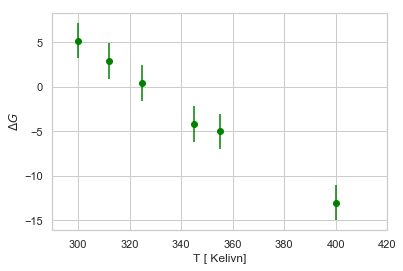We can use our equations above to find the entropy, which is the negative of the slope:

In :
cov_mat = np.cov(T, DG, ddof=1)
slope = cov_mat[0,1] / cov_mat[0,0]
DS = -slope
print(DS, 'kcal/mol*K')

0.18243128145567172 kcal/mol*K


Now if we want to give a confidence interval, we need to get the standard error first. Let's start by checking our fit and we'll need the residuals

In :
intercept = np.mean(DG - T * slope)
print(intercept)

plt.errorbar(T, DG, yerr=2, fmt='go', capthick=3)
plt.plot(T, T * slope + intercept, '-')
plt.xlim(290, 420)
plt.xlabel('T [ Kelivn]')
plt.ylabel('$\Delta G$')
plt.show()

59.652086720867224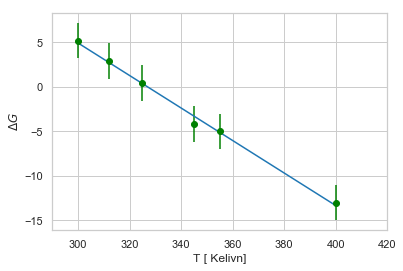In :
residuals = DG - T * slope - intercept
sig_e = np.sum(residuals**2) / (len(T) - 2)
#this is where we include the error in measurement
s2_e = sig_e + 2.0 ** 2
s2_slope = s2_e / (np.sum( (np.mean(T) - T)**2 ) )


Now we have the standard error in the slope, which is the same as entropy. Now we get our confidence interval

In :
T = scipy.stats.t.ppf(0.975, len(T) - 2)
slope_ci = T * np.sqrt(s2_slope)

print(slope_ci)

0.07134356636346217


Remember, the slope is the negative change in our entropy. So our final answer is

$$\Delta S = 0.18 \pm 0.06 \frac{\textrm{kcal}}{\textrm{mol}\cdot\textrm{K}}$$

# Case 2 - OLS with constant $x$ uncertainty in 1D¶

Now we have a measurement error in our independent variables. Our $x$ values are just our best esimiates; we don't know the true $x$ values. Our model equation is:

$$y = \alpha + \beta(x + \eta) + \epsilon$$

where our measurements are $y$ and $(x + \eta)$. Once again we can rearrange our model equation into:

$$y = \alpha + \beta x + \epsilon_2$$

where $\epsilon_2 = \beta \eta + \epsilon$. Everything is the same as before, except that our extra variance term depends on the slope. That changes our standard error equation to:

$$S^2_{\epsilon_2} = \frac{SSR}{N - 2} + \hat{\beta}^2\sigma_{\eta}^2$$

and $\sigma_{\eta}^2$ is again the squared error in our measurement. Note that this is an approximate method.

### All Equations for Case 2¶

$$\hat{\beta} = \frac{\sigma_{xy}}{\sigma_x^2}$$$$\hat{\alpha} = \frac{1}{N}\sum_i (y_i - \hat{\beta}x_i)$$$$S^2_{\epsilon_2} = \frac{SSR}{N-2} + \hat{\beta}^2\sigma_{\eta}^2$$$$S^2_{\alpha} = S^2_{\epsilon_2} \left[ \frac{1}{N-2} + \frac{\bar{x}^2}{\sum_i\left(x_i - \bar{x}\right)^2}\right]$$$$S^2_{\beta} = \frac{S^2_{\epsilon_2}}{\sum_i \left(x_i - \bar{x}\right)^2}$$

## Case 2 - Example¶

Repeat the case 1 example, except with an error in temperature measurement of 5 K.

In :
T = np.array([300., 312, 325, 345, 355, 400])
DG = np.array([5.2, 2.9, 0.4, -4.2,-5, -13])

plt.errorbar(T, DG, xerr=5, fmt='go', capthick=3)
plt.xlim(290, 420)
plt.xlabel('T [ Kelivn]')
plt.ylabel('$\Delta G$')
plt.show()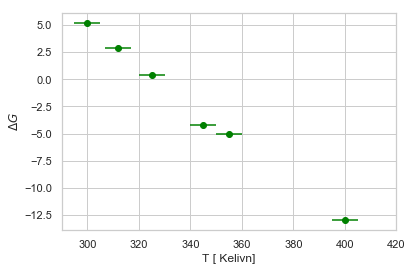Our slope and intercept are unchanged, but our standard error is different.

In :
#Now we use the independent variable measurement error
s2_e = sig_e + slope**2 * 5.0 ** 2
s2_slope = s2_e / (np.sum( (np.mean(T) - T)**2 ) )
T = scipy.stats.t.ppf(0.975, len(T) - 2)
slope_ci = T * np.sqrt(s2_slope)

print(slope_ci)

0.03616816441646756


With the new measurement error, our new confidence interval for entropy is:

$$\Delta S = 0.18 \pm 0.03 \frac{\textrm{kcal}}{\textrm{mol}\cdot\textrm{K}}$$

## Case 2 - Attenuation Error¶

There is an interesting side effect of independent measurement error. Let's look at some plots showing increasing uncertainty in $x$, but always with a slope of 3

In :
plt.figure(figsize=(24, 12))

rows = 2
cols = 3
N = 1000

for i in range(rows):
for j in range(cols):
index = i * cols + j + 1
fig = plt.subplot(rows, cols, index)
err = scipy.stats.norm.rvs(loc=0, scale = index - 1, size=N)
x = np.linspace(0,5, N)
y = 3 * (x + err)
plt.plot(x, y, 'o')
plt.xlim(-2, 7)
plt.title('$\sigma_\eta = {}$'.format(index))

plt.show()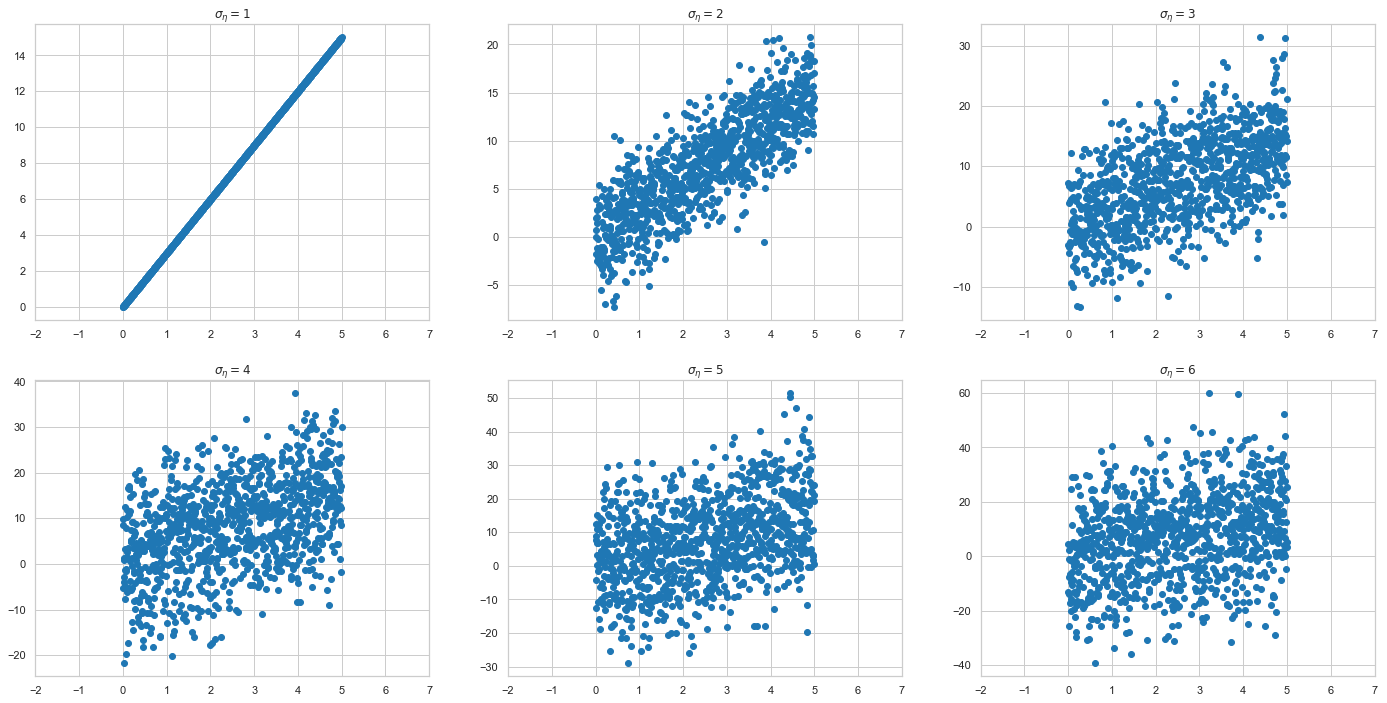Notice that as our error in our measurement gets larger, the slope becomes less and less clear. This is called Attenuation Error. As uncertainty in $x$ increases, our estimates for $\alpha$ and $\beta$ get smaller. We usually don't correct for this, because all our hypothesis tests become more conservative due to attenuation and thus we won't ever accidentally think there is a correlation when there isn't. But be aware that when the uncertatinty in $x$ becomes simiar in size to our range of data, we will underestimate the slope.

# Case 3 - OLS with constant x,y uncertainty in 1D¶

As you may have expected, the standard error in $\epsilon_3$ is just a combination of the previous two cases:

$$S^2_{\epsilon_3} = \frac{SSR}{N} + \hat{\beta}^2\sigma^2_{\eta_x} + \sigma^2_{\eta_y}$$

### All Equations for Case 3¶

$$\hat{\beta} = \frac{\sigma_{xy}}{\sigma_x^2}$$$$\hat{\alpha} = \frac{1}{N}\sum_i (y_i - \hat{\beta}x_i)$$$$S^2_{\epsilon_3} = \frac{SSR}{N - 2} + \hat{\beta}^2\sigma^2_{\eta_x} + \sigma^2_{\eta_y}$$$$S^2_{\alpha} = S^2_{\epsilon_3} \left[ \frac{1}{N - 2} + \frac{\bar{x}^2}{\sum_i\left(x_i - \bar{x}\right)^2}\right]$$$$S^2_{\beta} = \frac{S^2_{\epsilon_3}}{\sum_i \left(x_i - \bar{x}\right)^2}$$

## Case 3 - Example¶

Repeat the Case 1 example with an uncertainty in $\Delta G$ of 2 kcal/mol and $T$ of 5K

In :
temperature = np.array([300., 312, 325, 345, 355, 400])
DG = np.array([5.2, 2.9, 0.4, -4.2,-5, -13])

plt.errorbar(temperature, DG, xerr=5, yerr=2, fmt='go', capthick=3)
plt.xlim(290, 420)
plt.xlabel('T [ Kelivn]')
plt.ylabel('$\Delta G$')
plt.show()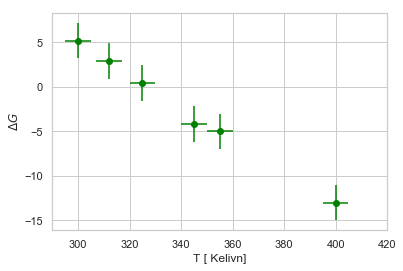In :
cov_mat = np.cov(temperature, DG, ddof=1)
slope = cov_mat[0,1] / cov_mat[0,0]
DS = -slope
print(DS, 'kcal/mol*K')
intercept = np.mean(DG - temperature * slope)
print(intercept)

plt.errorbar(temperature, DG, xerr=5, yerr=2, fmt='go', capthick=3)
plt.plot(temperature, temperature * slope + intercept, '-')
plt.xlim(290, 420)
plt.xlabel('T [ Kelivn]')
plt.ylabel('$\Delta G$')
plt.show()

0.18243128145567172 kcal/mol*K
59.652086720867224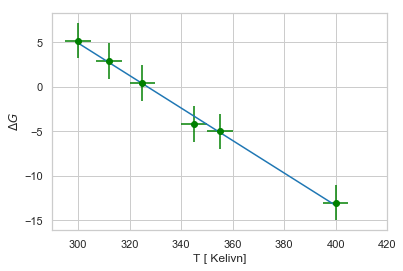In :
residuals = DG - temperature * slope - intercept
sig_e = np.sum(residuals**2)

#The only new part
#-------------------------------------
#Now we use both the dependent and the independent variable measurement error
sig_total = sig_e + slope**2 * 5.0 ** 2 + 2.0**2
#-------------------------------------

s2_e = sig_total / (len(temperature) - 2)
s2_slope = s2_e / (np.sum( (np.mean(temperature) - temperature)**2 ) )
T = scipy.stats.t.ppf(0.975, len(temperature) - 2)
slope_ci = T * np.sqrt(s2_slope)

print(slope_ci)

0.04191610991258184


With the both measurement errors, our confidence interval for entropy is:

$$\Delta S = 0.18 \pm 0.04 \frac{\textrm{kcal}}{\textrm{mol}\cdot\textrm{K}}$$

which is a slightly larger confidence interval than for case 1

# Case 4 - OLS with multiple y values in N-dimensions¶

Sometimes you'll see people have multiple measurements for each $y$-value so that they can plot error bars. For example, let's say we have 3 $x$-values and we have 4 $y$-vaules at each $x$-value. That would give enough samples so that we can compute a standard error at each $y$-value:

$$S_y = \sqrt{\frac{\sigma_y^2}{N}}$$
In :
x = np.array([2, 4, 6])

y_1 = np.array([1.2, 1.5, 1.1, 0.9])
y_2 = np.array([2.6, 2.2, 2.1, 2.5])
y_3 = np.array([3.0, 2.9, 3.3, 5])

y = np.array([np.mean(y_1), np.mean(y_2), np.mean(y_3)])
#compute standard error
yerr = np.sqrt(np.array([np.var(y_1, ddof=1), np.var(y_2, ddof=1), np.var(y_3, ddof=1)])) / 4.0

plt.errorbar(x, y, yerr=yerr, fmt='o', capthick=3)
plt.xlim(0, 10)
plt.show()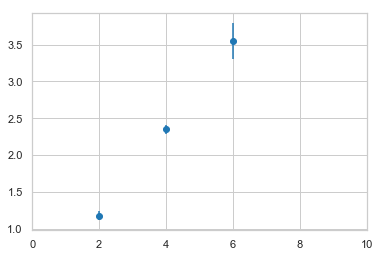How would we do regression on this? Well, it turns out you don't do anything special. You just treat the 12 $y$-values as equivalent and use the normal regression equations. I know this seems obvious, but it's a common question.